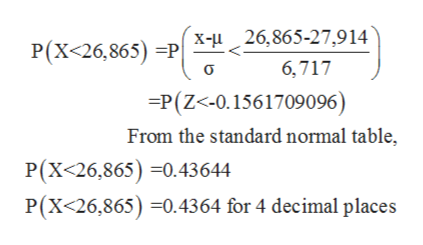# Private nonprofit four-year colleges charge, on average, \$27,914 per year in tuition and fees. The standard deviation is \$6,717. Assume the distribution is normal. Let X be the cost for a randomly selected college. Round all answers to 4 decimal places where possible.a. What is the distribution of X? X ~ N(,)b. Find the probability that a randomly selected Private nonprofit four-year college will cost less than 26,865 per year.c. Find the 60th percentile for this distribution. \$ (Round to the nearest dollar.)

Question
8 views

Private nonprofit four-year colleges charge, on average, \$27,914 per year in tuition and fees. The standard deviation is \$6,717. Assume the distribution is normal. Let X be the cost for a randomly selected college. Round all answers to 4 decimal places where possible.

a. What is the distribution of X? X ~ N(,)

b. Find the probability that a randomly selected Private nonprofit four-year college will cost less than 26,865 per year.

c. Find the 60th percentile for this distribution. \$ (Round to the nearest dollar.)

check_circle

Step 1

From the given information, mean (μ) = \$27,914 and standard deviation (σ) = \$6,717.

(a)

Given data normally distributed with mean \$27,914 and standard deviation \$6,717.

Therefore, distribution of ‘X’ is N~ (μ, σ)

∴ N~ (\$27,914, \$6,717)

Step 2

(b)

Let ‘X’ be a normal distribution random variable.

Now,

Probability that a randomly selected private nonprofit four year college will cost less than \$26,865 per year is,help_outlineImage Transcriptionclose26,865-27,914 X- P(X<26,865) P 6,717 -P(Z<-0.1561709096) From the standard normal table P(X<26,865) 0.43644 P(X<26,865) 0.4364 for 4 decimal places fullscreen
Step 3

Therefore, Probability that a randomly selected private nonprofit fou...

### Want to see the full answer?

See Solution

#### Want to see this answer and more?

Solutions are written by subject experts who are available 24/7. Questions are typically answered within 1 hour.*

See Solution
*Response times may vary by subject and question.
Tagged in

### Statistics